# On how EPR unifies Classical Theory with Quantum MechanicsLast Update April 5 2020

## Abstract

By 1935 Quantum Mechanics statistical interpretation of entangled particles were well established. Since then these statistics are often referred as probabilities in popular scientific articles and discussions. While statistics provide absolutely well defined that is certain outcomes, probabilities infer instead that these very outcomes might not occur at all, hence the uncertainty associated to Quantum Mechanics.
In the following the word probabilities is avoided, and the word statistics is systematically used instead.
By 1935 though these Quantum Statistics were contradicting Classical mathematics; and no experiments could be run that would confirm either theory.
That year Einstein, Podolsky, and Rosen, in a paper called EPR, demonstrated that hidden variables were underlying Quantum Mechanics statistics, in effect denying the uncertainty alledgedly tied to these statistics.
Almost thirty years later in 1964 John Stewart Bell in a disclosure now called "Bell's Inequalities" challenged EPR.
Bell's inequalities stated that should some physical experiments show that Quantum statistics prevail there would be no hidden variables.
Alain Aspect experiments in the 1980's definitely justified Quantum Mechanics theory.
As a consequence in science, uncertainty became fact of life, and since then EPR's hidden variables are decried.

Reversing this trend, in 2017 this author uncovered the hidden, yet evident variable underlying Quantum entanglement statistics; this web-page provides a classical or local equation describing explicitly the phenomenon, proving EPR right after all.
Beware, the following is considered to be wrong, and simply disregarded, by most professional physicists.

## 1. Introduction

Quantum mechanics is defining, studying and experimenting physically entangled pairs of electrons and photons. Amazingly nobody knows about the materialistic reality that is the physical constitution of either the electron or the photon particle.
Nevertheless physicists accomplished breathtaking measures and made astonishing discoveries concerning these entangled pairs.
First, in Quantum mechanics, the 2 particles of any entangled pair are identical twins; all entangled pairs are furthermore identical, all entangled pairs occur under a common unique and identical format, which is independent of the particles characteristics.
This common and universal format, called Quantum State, occurs under a number of various eventual yet incompatible occurrences, which justify the uncertainty attached to it.
Rather than relying on explicit measures and mathematics, which handles known variables as done in Classical Physics, Quantum Mechanics is relying on quantum state and statistics, and as already mentionned often called probabilities in the press.

## 2. Classical Explicit Interpretation and Predictions, as interpreted by science

In this section I am leaving the Quantum World made of statistics and I am describing instead the explicit aspect of entangled pairs as interpreted in science.
In classical physics the particles involved are polarized, having a magnetic North Pole pointing or oriented in any direction within the full spectrum extending from 00 to 3600 (read zero degree to three hundred and sixty degrees); these angular orientations are explicitly, that is individually, detected and measured.
And to emphasize the discripency between Quantum and Classical Physics these angular orientations are ignored in Quantum Mechanics. In Quantum Mechanics these angular orientations are irrelevant and do not exist until the measures occur at which time they become suddenly measurable and well defined.
Now getting back to Classical Physics, the two tilted red-green bars labeled with an "n" (for North Pole) of Figure 1 represent two particles of an entangled pair explicitly oriented at 600. The 2 particles have been emitted from the Emitter represented in the center; the left particle is travelling toward the left, the right particle toward the right, both remaining oriented at 600 until measured.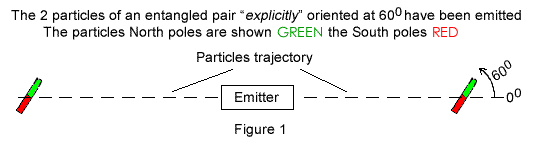Figure 2 illustrates the experiment's fundamental features; two detectors are set on either sides of the Emitter; these detectors are polarized entities akin to magnets represented here by colored circles with a diameter separating the North Pole (N) half circumference represented in GREEN from the South (S) pole represented in RED. The Left Detector is oriented at ΘLD = 00 (read theta LD) while the Right Detector is oriented at ΘRD = 1200. When a particle's North Pole matches its detector's North Pole (in GREEN) as illustrated in left of Figure 2, the detector should be flashing a GREEN signal. When the particle's and detector's North pole do not match, as is the case in the Right Detector, a RED signal should be flashed.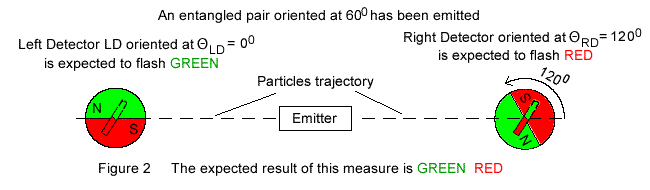The words "should be flashing" and "expected to flash" have been used because, as shown in the following, this explicit reasoning turns out to contradict both Quantum Mechanics predictions and physical experiments.

Having in mind that the 2 particles of any pair have identical orientation, Figure 3 shows that all of the pairs oriented from 00 to 1800 should flash GREEN in the Left Detector.
Yet the pairs oriented from 00 to 1200, or two thirds of the top half circle should flash RED in the Right Detector. Only the pairs oriented from 1200 to 1800, or one third of the top half circle should flash GREEN in the Right Detector.
All in all only one third of the pairs between 00 and 1800 should flash identical GREEN signals, two thirds should flash different GREEN / RED signals
And for the pairs oriented from 1800 to 3600 one third should flash identical RED signals, two thirds should flash different RED / GREEN signals.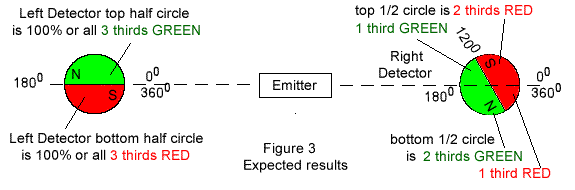In this Classical explicit interpretation, one third of the pairs should flash identical colors; two thirds of the pairs should flash differing colors.
This text is in red as experiments ran later proved this explicit reasoning to be wrong!

Because this explicit reasoning turns out to be wrong, these angular orientations are considered to be non existant in Quantum mechanics and the entangled pair's quantum state does not involve these orientations.
Note that these explicit angular orientations are expressed in a statistical format involving population's thirds. This is done on purpose, it allows for the comparison between this explicit interpretation and Quantum statistical interpretation done in the next section.

## 3. On how Quantum Mechanics Contradicts Classical Explicit Predictions

Should mathematics not be your bag, simply ignore Equation 1 and jump to the paragraph printed in green color below.
In the early 20th century Quantum mechanics following Equation providing statistical information had been set: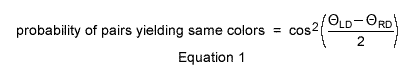The detectors orientations ΘLD and ΘRD can be each given any values from 00 to 3600; Quantum Mechanics Equation 1 provides the probability of pairs yielding same and differing colors.

When the detectors angles are set at ΘLD = 00 and ΘRD = 1200, as in Figures 2 and 3, Quantum mechanics (Equation 1) predicts that same colors (either GREEN GREEN or RED RED) will be flashed one-quarter of the times, instead of one-third of the time, and opposite colors (either RED GREEN or GREEN RED) will be flashed three-quarters of the time, instead of two-thirds of the time.
This text is in green as experiments ran later proved this Quantum Statistics to fit reality.

These statistical predictions are definitely contradicting the explicit outcome just described in section 2. above.
By 1925 both the Classical explicit outcome (section 2. above) and this contradictory Quantum Mechanics statistical prediction were well established.
No physical experiments were yet performed though; nobody knew whether the explicit explanation or this Equation 1 contradictory statistics would be confirmed by experiments.

## 4. How the Quantum Statistics Get Justified Beforehand

The first fundamental aspect of above predictions is that should Quantum Mechanics prevail, explicit reasoning would become wrong; Figure 3 distributions and explicit reasoning would all become irrelevant.
Because the explicit theory would now contradict reality, uncertainty would then rule.

Furthermore in order to depart from our human explicit reasoning, the two particles of any pair would have to communicate and instruct each other how to behave instantly over distance at time of measure in order to comply; and such instantaneous communication contradicts Einstein's relativity in which no signal can be transferred faster than light.
This aspect of Quantum Mechanics has been given the name of nonlocality.

Facing such novelties, Einstein, Podolsky, and Rosen came up in 1935 with their famous paper now called EPR demonstrating that behind Quantum statistics, there are hidden variables that explain the particles' individual behaviors.
While justifying Quantum mathematics statistical results, EPR ends up challenging both uncertainty and nonlocality. Please note that EPR does not deny quantum mechanics mathematics and statistics, EPR is denying only the language attached to it, namely the words uncertainty and nonlocality.

## 5. The Experiments and Consequences

During a number of years no experiments were run that could determine whether Quantum statistics or explicit mathematics would rule. Both theories were still plausible. When one of the two would take over the mathematical validity of the other would have to be disqualified.
In 1964 John Stewart Bell took the initiative; he came up with his famous inequalities, establishing that should future physical experiments justify Quantum Mechanics statistical predictions, the explicit explanation (section 2. above) would not only become irrelevant, but in addition no explicit explanation could be found; there would be no explicit explanation at all.

The first physical experiments and undisputable measures that occurred in the 1980's definitely showed that Quantum statistics were ruling over the explicit explanation.
Now according to Bell's famous Inequalities, which has been the consensus for over the next 50 years, not only our human explicit interpretation is wrong, but no hidden variables should ever be uncovered.

## 6. New Explicit Mathematics Complying with Experiments and Substantiating EPR

In 2017 I showed that explicit Mathematics Equation 2 (below) is outperforming Quantum Mechanics achievement in that, besides conforming to Quantum statistics, it brings to light the individual behavior of each particle, a feast banished by Bell's theorem.
Once more beware, the following language and equation is denied by most professional physicists; those I contacted simply abnegate the following, even refusing to look at it.
For instance the following equation and explanations have been brought to a physics professor's attention, who replied that angle orientations do not exist in the quantum world (period); think of it, one must conclude that entangled pairs, somehow are occurring in a "real world" that is "non existing".

In Equation 2 the particles' reorientations bring to mind the refraction phenomenon, which involves the deflection of the appearance of a rod partly immerged under water; the words reorientation and reoriented, rather than refraction and refracted, have been specifically used in this web page to point out that the pheonomenon studied is not refraction.

Provided the pairs are evenly distributed when emitted, those with tilts between 00 and 1350 represent three quarters of those between 00 and 1800. The remaining pairs between 1350 and 1800 make the remaining quarter. And an identical distribution applies to the circumference's lower half.
In order to match the physical measures and Quantum Mechanics statistics when the detectors are set 1200 apart, it suffices to come up with an equation that modifies the pairs tilted between 00 and 1350 to angles tilted between 00 and 1200. This reorientation occurs as follows: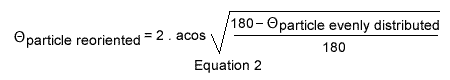Equation 2 contradicts Bell's inequalities, which states that no explicit explanation can be given.

When the detectors are oriented 1200 apart as in Figure 3, the particles evenly tilted from 00 to 1350 when emitted will be, according to Equation 2, reoriented and measured between 00 and 1200 by both Detectors; these explicit reorientations precisely coincide with the physical measures and Quantum mechanics statistics.
This explicit Interpretation is written in green because it is in agreement with both experiments and Quantum Mechanics statistics.

In this interpretation the 2 particles of any pair are acting in concert, not because one particle is instructing the other to comply instantly over distance (Quantum Mechanics nonlocality interpretation), but because, before the measures take place, their individual yet common tilts have each been re-oriented in an equal amount as provided by Equation 2.
Please note that the 00, 1200 detectors setting as used in all of the above, is not restrictive; the 2 detectors can be set at any of two values other than 00 and 1200; in all cases explicit mathematics Equation 2 provides reoriented values that fit the experiments, just as Quantum mechanics Equation 1 does.
Above section 2. former wrong explicit one third two thirds distribution used by Bell and not conforming to reality is abolished right here and replaced by Equation 2 correct explicit one quarter three quarters distribution and conforming to reality and Quantum statistics.

## 7. Computer Simulation

The explicit mathematical Equation 2 predicts the exact behavior of each particle of each pair, a break actually forbidden by Bell's famous inequalities.
Whereas Quantum Mechanics provides the overall correct distribution of the measures at once, that is through a single calculation using Equation 1, the explicit Equation 2 requires much more work:
1. Equation 2 requires 5 mathematical operations to find out the reorientation of a single particle; and these five operations would have to be successively repeated a great number of times on a great number of particles to be chosen evenly distributed over the 3600 spectrum.
2. Finally besides these cumbersome calculations, each calculated reorientation would have to be confronted to both detectors and be subsequently compiled to verify that the results provided by Equation 2 agree with Quantum Mechanics Equation 1 statistical results.
A computer simulation is of the essence; one has been set; anyone can trigger and use it; for instance one can choose to set the angles of the two detectors 1200 apart and choose to emit 360 pairs; the program displays the individual 360 particles tilts when emitted and their respective reoriented angles over the full circumference along with their equivalent statistics, the later conforming to Quantum Mechanics statistics. Just right click this link and "open in new tab" : Entanglement Computer Simulation.
The computer simulation led to the discovery that the 2 electrons of a pair must be individually polarized by their respective detectors when entering the latter, the whole process being resumed as follows:
• First the electrons are individually polarized by, and in the amount of, their respective detectors orientations. This is providing to the 2 electrons of any pair an equal orientation, or aligning them yet taking in account their respective orientations when emitted. That amounts at relating the two electrons behaviors, in accordance with each other as in Quantum Mechanics; yet because it is also taking in account the respective detectors' orientations, the whole process replaces Quantum Mechanics alleged mutual interaction over distance.
• Then the individual diffractions are occuring on each side again individually by their own detectors.

The reorientations shown Figure 4 have been attained using above application; it illustrates explicit mathematics Equation 2; and as just mentioned, because the 2 particles of any pair when measured have been given identical orientations (through their individual polarizations), each red and green lines in Figure 4 represent a pair orientation as well as each particle's orientation of that pair. An assertion that can be verified running the computer simulation proposed above.Important note about the computer simulation that I designed and I am proposing.
I have been told that the computer program I wrote is concerning a specific case, and not the general phenomenon. My answer is that my program precisely simulates Alain Aspect experiment, which is the quintessential case on which all of Quantum Mechanics is based.
Then I must mention the following.
Someone told me, you can design whatever you please using a computer language or code. And that is true, using Quantum Mechanics above equation 1, the computer code will provide the right results.
So I must concede the following:
When the detectors are respectively set at 0 and 120 degrees, local reality equation 2 independently applied to each electron (no interaction at distance between the 2 simultaneous measures is occorring) provides one quarter three quarter distribution. Showing clearly LOCAL-REALITY is in force.
Unfortunately I have been unable to this day (April 2020) to make computer code provide the same results (one quarter three quarter distribution) for other settings than A = 0 B = 120, such as A = 30 degrees B = 150 degrees for instance.
In such cases (other than A = 0, B = 120) my PHP code is taking in account the difference between the 2 detectors to come up with the right outcome; this means that in practically all other cases (other than A = 0, B = 120) I have to take in account non-locality (Quantum Mechanics logic, that is the angular difference between the 2 detectors) along local-reality equation 2 in order to provide the correct answer.

## 8. Conclusion

The ultimate goal is to refute both uncertainty and nonlocality concepts, while appreciating Quantum Mechanics statistical interpretation.
Another goal is to provide as best as possible a physical explanation of the phenomenon as done above.

Statistics does not mandate uncertainty
Equation 2, which predicts precisely the particles' individual behaviors, abolishes the uncertainty associated to Quantum Equation 1 statistics.
And that is going along EPR's hidden variables that disavow uncertainty without denying Quantum statistics.

Nonlocality is unreasonably commended
When the detectors are set 1200 apart, the mathematics as well as the experiments show that one quarter of the pairs are providing differing colors.
Nonlocality, which states that when one particle is measured the other complies at distance, then cannot refer to the colors measured. Nonlocality must then refer to the fact that the statistics do not coincide to the expected outcome; nonlocality is a byproduct of unexplained statistics and vanishes with Equation 2.

Bell's inequalities revisited
Based on the wrong one third same colors to two thirds different colors distribution, Bell's famous inequalities are simply dismissed. Please note that Bell's mathematics are certainly right. This author thinks that Bell's conclusion is wrong, not because its mathematics is faulty, but simply because it initialy rests on this 1 third /3 thirds distribution wrong hypothesis.

EPR's hidden variables reinstated
This interpretation has the definite advantage to confirm that Einstein and colleagues mathematics is right after all. The hidden variable is evidently the particles individual orientations.

From a purely logical point of view, should uncertainty rule it would mean that uncertainty is uncertain or unreliable... uncertainty cannot rule; on the other hand certainty, a very plausible alternative, allows itself to rule.
And from a very practical point of view please note that quantum uncertainty always ends up to be metamorphosed into local or explicit certainty that is Classical Physics.
An official objection to above quantum scale certainty rehabilitation, is that there is no angle orientation in the quantum world; but that objection does not hold for several reasons: first at emission the particles orientations have to be evenly distributed; suppose the particles orientations at emission are restrained between zero and ninety degrees, the measures will not coincide to quantum statistics; in short the particles orientations are of the essence right at emission; then in quantum theory the 2 particles of a pair are complementary, which means that they are of opposite directions and that involves the particles directions before the measure takes place; finally the measures themseves not only involve but are all about individual particles' orientations. Contradicting the arguments of those who are advocating uncertainty, the particle's orientations permeate quantum mathematics throughout and are integral part of the quantum world and reality.
As a final note, while we know with certainty that life on Earth has a finite time span, meaning that we know with certainty that we will die, we human are nevertheless not given the ability to precisely predict all aspects of our own future. In spite of the Natural Laws, that among other rule with certainty over entangled pairs, our human brain can only be uncertain about many other things.

## References

 Einstein, A., Podolsky, B., Rosen, N.: Can quantum-mechanical description of physical reality be considered complete?
Phys. Rev. 47, 777-780 (1935).
Also available on internet at: EPR
 Bell, J.S.: On the Einstein Podolsky Rosen paradox. Phys. 1, 195-200 (1964)
Available on internet at: Bell's inequalities
[2 a] For everybody explanation: Bell's inequalities for everybody
 Aspect, A., Grangier, P., Roger, G.: Experimental realization of Einstein-Podolsky-Rosen-Bohm Gedanken experiment: a new violation of Bell's inequalities. Phys. Rev. Lett. 49, 91-94 (1982) Available on Internet: Alain Aspect experiments
 American Journal Of Physics; Volume 49; Number 10; Page 940; October 1981.
Bringing home the atomic world: Quantum mysteries for anybody. By N.D.Mermin.
Also available on internet: Mermin's paper
 See On how the 33% distribution is set ignoring the particles orientations
 Quote found Dec 19 2019 on Wikipedia at Wikipedia, the free encyclopedia title: Bell's theorem
 Quote also found Dec 19 2019 on Wikipedia at Wikipedia, the free encyclopedia title: Quantum entanglement

## Appendix A: Quantum Mechanics hidden side

This section sheds light on Quantum Mechanics Equation 1 extraordinary alchemy.
It turns out that the origin chosen to refer the detectors orientations is irrelevant. We can then choose Detector B as being the reference in Equation 1, which can then be rewritten as: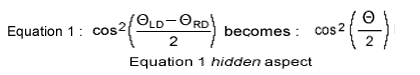Both Equation 1 and its hidden aspect deal with the detectors' orientations rather than with the electrons tilts as does Equation2; the hidden side of Equation 1 suggests that detectors' orientations just as electrons' tilts can be re-oriented in order to describe the entanglement phenomenon. Equation 3, which is inspired by above hidden aspect of Equation 1, does just that:Equation 3 modifies θDetectorNominalOrientation = 1200 into θDetectorEffectiveOrientation = 1350 and θDetectorNominalOrientation = 00 remains unchanged at θDetectorEffectiveOrientation = 00.
In these conditions one quarter identical measures instead of one third identical measures will occur, provided the emitted electrons are evenly distributed over the full 3600 circumference.
In the end Quantum Mechanics extraordinary alchemy mends nicely with local reality.

Contact:   henriJMsalles[at]gmail.com
Copyright © by Henri Salles. You have the permission to reproduce, print, distribute and post the contents of this webpage, provided you mention the link: epr-substantiation.info by Henri Salles